Test: Electrical Machines- 4

# Test: Electrical Machines- 4

Test Description

## 10 Questions MCQ Test GATE Electrical Engineering (EE) 2023 Mock Test Series | Test: Electrical Machines- 4

Test: Electrical Machines- 4 for Electrical Engineering (EE) 2023 is part of GATE Electrical Engineering (EE) 2023 Mock Test Series preparation. The Test: Electrical Machines- 4 questions and answers have been prepared according to the Electrical Engineering (EE) exam syllabus.The Test: Electrical Machines- 4 MCQs are made for Electrical Engineering (EE) 2023 Exam. Find important definitions, questions, notes, meanings, examples, exercises, MCQs and online tests for Test: Electrical Machines- 4 below.
Solutions of Test: Electrical Machines- 4 questions in English are available as part of our GATE Electrical Engineering (EE) 2023 Mock Test Series for Electrical Engineering (EE) & Test: Electrical Machines- 4 solutions in Hindi for GATE Electrical Engineering (EE) 2023 Mock Test Series course. Download more important topics, notes, lectures and mock test series for Electrical Engineering (EE) Exam by signing up for free. Attempt Test: Electrical Machines- 4 | 10 questions in 30 minutes | Mock test for Electrical Engineering (EE) preparation | Free important questions MCQ to study GATE Electrical Engineering (EE) 2023 Mock Test Series for Electrical Engineering (EE) Exam | Download free PDF with solutions
 1 Crore+ students have signed up on EduRev. Have you?
Test: Electrical Machines- 4 - Question 1

### A short shunt compound wound motor is having series field resistance, armature resistance and shunt field resistance of 0.6 Ω, 0.4 Ω and 250 Ω respectively. If the motor is drawing 50 A at 500 V, what the voltage drop across series field resistance?

Detailed Solution for Test: Electrical Machines- 4 - Question 1

Short shunt motor be like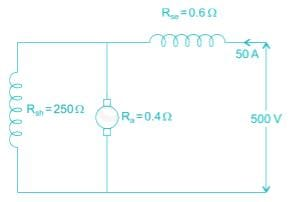Voltage drop across series field resistance
= 50 × 0.6
= 30 V

Test: Electrical Machines- 4 - Question 2

### A DC shunt motor of 200 V, 10.5 A, 2000 rpm has an armature resistance of 0.5 Ω and field resistance of 400 Ω. It drives a load whose torque is constant at rated motor torque. What is the value of armature current if the source voltage drops to 175 V?

Detailed Solution for Test: Electrical Machines- 4 - Question 2

Given V1 = 200 V
Armature resistance (Ra) = 0.5 Ω
Field winding resistance (Rsh) = 400 Ω
Field current = 200/400 = 0.5 A
Armature current = 10.5 – 0.5 = 10 A
Given that load torque is constant.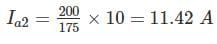*Answer can only contain numeric values
Test: Electrical Machines- 4 - Question 3

### A six pole, 60 kW DC machine operating at 1200 rpm has a generated emf of 150 V. If the speed is reduced to 70% of its original value and the pole flux is tripled, then induced emf would be___ (in V)

Detailed Solution for Test: Electrical Machines- 4 - Question 3

In a DC machine,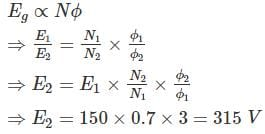Test: Electrical Machines- 4 - Question 4

A long shunt compound wound motor is having shunt field resistance, series field resistance and armature resistance of 300 Ω, 0.8 Ω and 0.6 Ω respectively. If the motor is drawings 60 A at 500 V, what is the voltage drop across series field resistance?

Detailed Solution for Test: Electrical Machines- 4 - Question 4

Long shunt motor circuit is given as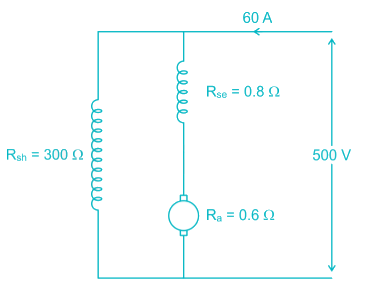Current through shunt field = 500/300 = 1.67 A
∴ Armature current Ia = 58.33A
Voltage across series field resistance
= 58.33 × 0.8
= 46.664 V

*Answer can only contain numeric values
Test: Electrical Machines- 4 - Question 5

A 220 V DC shunt motor takes 3 A at no-load. It draws 25 A when running at full-load at 1500 rpm. The armature and shunt resistances are 0.5 Ω and 220 Ω, respectively. The no- load speed in rpm (round off to two decimal places) is ________.

Detailed Solution for Test: Electrical Machines- 4 - Question 5

Voltage (V) = 220 V
No load current (INL) = 3 A
Full load current (IFL) = 25 A
Full load speed (NFL) = 1500 rpm
Armature resistance (Ra) = 0.5 Ω
Shunt resistance (Rsh) = 220 Ω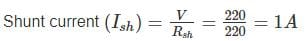Armature current (Ia) = IL - Ish
When motor is operating at no load
IanL = INL - Ish = 3 – 1 = 2 A
Eb1 = V - IanL Ra
= 220 – (2) (0.5) = 219 V
When motor is operating at full load
IaFL = IFL - Ish = 25 – 1 = 24 A
Eb2 = V – IaFL Ra
= 220 – (24) (0.5) = 208 V
We know that, in a DC shunt motor
Eb α N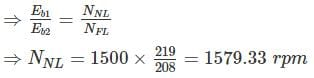*Answer can only contain numeric values
Test: Electrical Machines- 4 - Question 6

A 600 V series motor of resistance 1 Ω runs at 800 rpm when the current is 60 A. If an identical motor is mechanically coupled and joined in series, what will be the current (in A) when the set runs at 400 rpm and exerts twice the original gross torque?

Detailed Solution for Test: Electrical Machines- 4 - Question 6

Eb1 = V − IaRa = 600 − 1 × 60 = 540
Let the current when joined in in series = Ia2
The resistance = 2 × 1 = 2 Ω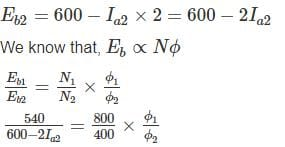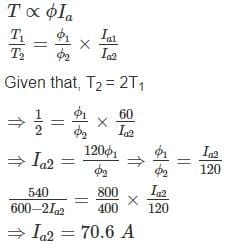*Answer can only contain numeric values
Test: Electrical Machines- 4 - Question 7

A 250-V DC shunt motor has a shunt field resistance of 150 Ω and an armature resistance of 0.3 Ω. For a given load. The motor runs at 1400 rpm, and drawing 20 A current. If a resistance of 75 Ω is added in series with the field, find the new armature current (in A). Assume load torque and flux and constant.

Detailed Solution for Test: Electrical Machines- 4 - Question 7

The field current corresponds to: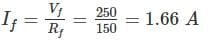Now:
IA = IL - If = 20 – 1.66 = 18.34 Amps
Observe that the current of the field circuit is much smaller than the armature current. Thus, adding the speed control resistance leads to minor power dissipation.

Ea = VS – IaRa = 250 – 5.502 = 244.5 V

EA = K ϕ ω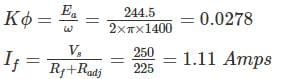The new armature current corresponds to:
Ia = I– If = 20 – 1.11 = 18.89 Amps

*Answer can only contain numeric values
Test: Electrical Machines- 4 - Question 8

A separately excited DC generator, when running at 1200 rpm supplies 200 A at 125 V to a circuit of constant resistant what will be the current (in amp) when the speed is dropped to 1000 rpm and the field is reduced to 80%. Armature resistance is 0.04 Ω and total drop at brushes is 2V.

Detailed Solution for Test: Electrical Machines- 4 - Question 8

Eg1 = VL + IaRa + 2 = 125 + (0.04 × 200) + 2 = 135 V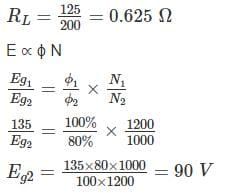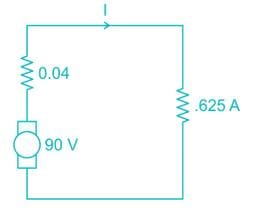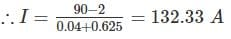*Answer can only contain numeric values
Test: Electrical Machines- 4 - Question 9

A 440 V DC shunt motor is running at 1000 RPM at full load torque, reduced armature voltage and full field. If load torque is reduced to 50% of rated value with armature voltage and field voltage held constant at previous value, the speed increase 1050 rpm. Find armature voltage drop (in V) at full load. Neglect effect of armature reaction. [Take no load speed 1000 rpm]

Detailed Solution for Test: Electrical Machines- 4 - Question 9

Ea1 = (440 – Ia1ra)
Ea1 = k ϕ1 ω1
Motor torque T1 = kaϕ1Ia1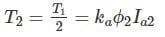As field voltage held constant ϕ2 = ϕ1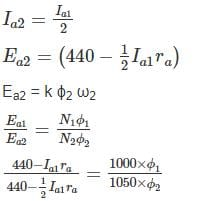440 × 21 – 21 Ia1r = 440 × 20 – 10 Ia1ra

∴ Armature voltage drop Ia1ra = 440/11 = 40 V

*Answer can only contain numeric values
Test: Electrical Machines- 4 - Question 10

A 400 V dc shunt motor takes a current of 6.2 A on no load and 70.4 A on full - load. Armature reaction weakens the field by 4%. Calculate the ratio of full-load speed to no-load speed. Given Ra = 0.20 Ω, Brush voltage drop = 2 V, Rf = 200 Ω.

Detailed Solution for Test: Electrical Machines- 4 - Question 10

Field current If  = 400/200 = 2 A
Iao = 6.2 – 2 = 4.2 A
Eao = 400 – 0.20 × 4.2 – 2 = 397.16 V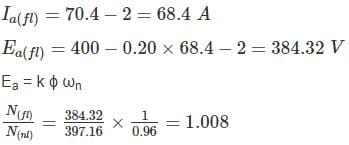## GATE Electrical Engineering (EE) 2023 Mock Test Series

22 docs|274 tests
Information about Test: Electrical Machines- 4 Page
In this test you can find the Exam questions for Test: Electrical Machines- 4 solved & explained in the simplest way possible. Besides giving Questions and answers for Test: Electrical Machines- 4, EduRev gives you an ample number of Online tests for practice

## GATE Electrical Engineering (EE) 2023 Mock Test Series

22 docs|274 tests(Scan QR code)